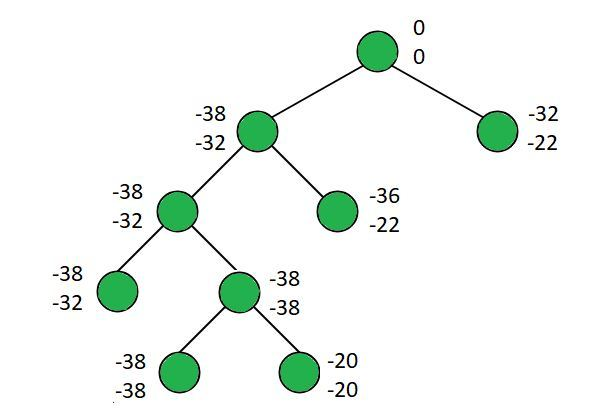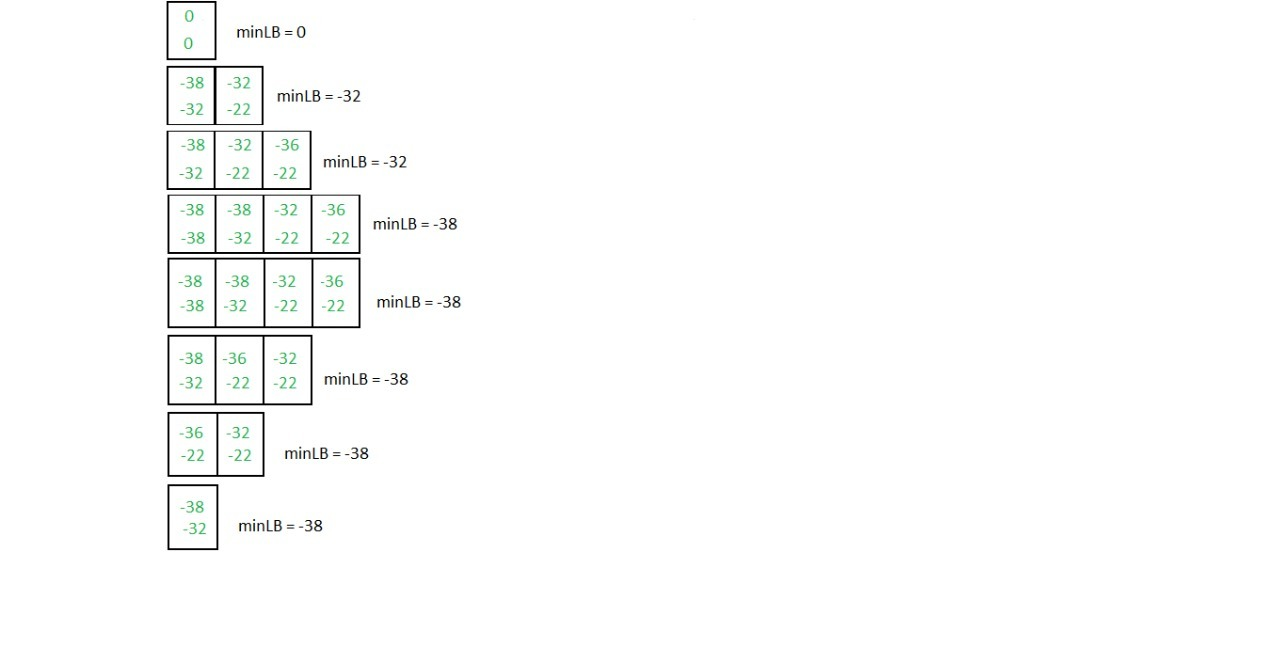# 0/1 Knapsack using Least Cost Branch and Bound

• Difficulty Level : Hard
• Last Updated : 01 Feb, 2022

Given N items with weights W[0..n-1], values V[0..n-1] and a knapsack with capacity C, select the items such that:

1. The sum of weights taken into the knapsack is less than or equal to C.
2. The sum of values of the items in the knapsack is maximum among all the possible combinations.

Examples:

Input: N = 4, C = 15, V[]= {10, 10, 12, 18}, W[]= {2, 4, 6, 9}
Output:
Items taken into the knapsack are
1 1 0 1
Maximum profit is 38
Explanation:
1 in the output indicates that the item is included in the knapsack while 0 indicates that the item is excluded.
Since the maximum possible cost allowed is 15, the ways to select items are:
(1 1 0 1) -> Cost = 2 + 4 + 9 = 15, Profit = 10 + 10 + 18 = 38.
(0 0 1 1) -> Cost = 6 + 9 = 15, Profit = 12 + 18 = 30
(1 1 1 0) -> Cost = 2 + 4 + 6 = 12, Profit = 32
Hence, maximum profit possible within a cost of 15 is 38.
Input: N = 4, C = 21, V[]= {18, 20, 14, 18}, W[]= {6, 3, 5, 9}
Output:
Items taken into the knapsack are
1 1 0 1
Maximum profit is 56
Explanation:
Cost = 6 + 3 + 9 = 18
Profit = 18 + 20 + 18 = 56

Approach:
In this post, the implementation of Branch and Bound method using Least cost(LC) for 0/1 Knapsack Problem is discussed.
Branch and Bound can be solved using FIFO, LIFO and LC strategies. The least cost(LC) is considered the most intelligent as it selects the next node based on a Heuristic Cost Function. It picks the one with the least cost.
As 0/1 Knapsack is about maximizing the total value, we cannot directly use the LC Branch and Bound technique to solve this. Instead, we convert this into a minimization problem by taking negative of the given values.
Follow the steps below to solve the problem:

1. Sort the items based on their value/weight(V/W) ratio.
2. Insert a dummy node into the priority queue.
3. Repeat the following steps until the priority queue is empty:
• Extract the peek element from the priority queue and assign it to the current node.
• If the upper bound of the current node is less than minLB, the minimum lower bound of all the nodes explored, then there is no point of exploration. So, continue with the next element. The reason for not considering the nodes whose upper bound is greater than minLB is that, the upper bound stores the best value that might be achieved. If the best value itself is not optimal than minLB, then exploring that path is of no use.

• Update the path array.
• If the current node’s level is N, then check whether the lower bound of the current node is less than finalLB, minimum lower bound of all the paths that reached the final level. If it is true, update the finalPath and finalLB. Otherwise, continue with the next element.
• Calculate the lower and upper bounds of the right child of the current node.
• If the current item can be inserted into the knapsack, then calculate the lower and upper bound of the left child of the current node.
• Update the minLB and insert the children if their upper bound is less than minLB.

Illustration:
N = 4, C = 15, V[]= {10 10 12 18}, W[]= {2 4 6 9}Left branch and right branch at ith level stores the maximum obtained including and excluding the ith element.
Below image shows the state of the priority queue after every step:Below is the implementation of the above approach:

## C++

 `// C++ Program to implement 0/1``// knapsack using LC Branch and Bound` `#include ``using` `namespace` `std;` `// Stores the number of items``int` `size;` `// Stores the knapsack capacity``float` `capacity;` `typedef` `struct` `Item {` `    ``// Stores the weight of items``    ``float` `weight;` `    ``// Stores the value of items``    ``int` `value;` `    ``// Stores the index of items``    ``int` `idx;``} Item;` `typedef` `struct` `Node {` `    ``// Upper Bound: Best case``    ``// (Fractional Knapsack)``    ``float` `ub;` `    ``// Lower Bound: Worst case (0/1)``    ``float` `lb;` `    ``// Level of the node``    ``// in the decision tree``    ``int` `level;` `    ``// Stores if the current item is``    ``// selected or not``    ``bool` `flag;` `    ``// Total Value: Stores the sum of the``    ``// values of the items included``    ``float` `tv;` `    ``// Total Weight: Stores the sum of the``    ``// weights of the items included``    ``float` `tw;``} Node;` `// Function to calculate upper bound``// (includes fractional part of the items)``float` `upper_bound(``float` `tv, ``float` `tw,``                  ``int` `idx, vector& arr)``{``    ``float` `value = tv;``    ``float` `weight = tw;``    ``for` `(``int` `i = idx; i < size; i++) {``        ``if` `(weight + arr[i].weight``            ``<= capacity) {``            ``weight += arr[i].weight;``            ``value -= arr[i].value;``        ``}``        ``else` `{``            ``value -= (``float``)(capacity``                             ``- weight)``                     ``/ arr[i].weight``                     ``* arr[i].value;``            ``break``;``        ``}``    ``}``    ``return` `value;``}` `// Function to calculate lower bound (doesn't``// include fractional part of the items)``float` `lower_bound(``float` `tv, ``float` `tw,``                  ``int` `idx, vector& arr)``{``    ``float` `value = tv;``    ``float` `weight = tw;``    ``for` `(``int` `i = idx; i < size; i++) {``        ``if` `(weight + arr[i].weight``            ``<= capacity) {``            ``weight += arr[i].weight;``            ``value -= arr[i].value;``        ``}``        ``else` `{``            ``break``;``        ``}``    ``}``    ``return` `value;``}` `class` `comp {``public``:``    ``bool` `operator()(Node a, Node b)``    ``{``        ``return` `a.lb > b.lb;``    ``}``};` `void` `assign(Node& a, ``float` `ub, ``float` `lb,``            ``int` `level, ``bool` `flag,``            ``float` `tv, ``float` `tw)``{``    ``a.ub = ub;``    ``a.lb = lb;``    ``a.level = level;``    ``a.flag = flag;``    ``a.tv = tv;``    ``a.tw = tw;``}` `void` `knapsack(vector& arr)``{` `    ``// Sort the items based on the``    ``// profit/weight ratio``    ``sort(arr.begin(), arr.end(),``         ``[&](Item& a, Item& b) {``             ``return` `a.value / a.weight``                    ``> b.value / b.weight;``         ``});` `    ``// min_lb -> Minimum lower bound``    ``// of all the nodes explored` `    ``// final_lb -> Minimum lower bound``    ``// of all the paths that reached``    ``// the final level``    ``float` `min_lb = 0, final_lb = INT_MAX;` `    ``// curr_path -> Boolean array to store``    ``// at every index if the element is``    ``// included or not` `    ``// final_path -> Boolean array to store``    ``// the result of selection array when``    ``// it reached the last level``    ``bool` `curr_path[size], final_path[size];` `    ``// Priority queue to store the nodes``    ``// based on lower bounds``    ``priority_queue,``                   ``comp>``        ``pq;` `    ``Node current, left, right;``    ``current.lb = current.ub = current.tw``        ``= current.tv = current.level``        ``= current.flag = 0;` `    ``// Insert a dummy node``    ``pq.push(current);` `    ``for` `(``int` `i = 0; i < size; i++)``        ``curr_path[i] = final_path[i]``            ``= ``false``;` `    ``while` `(!pq.empty()) {``        ``current = pq.top();``        ``pq.pop();` `        ``if` `(current.ub > min_lb``            ``|| current.ub >= final_lb) {` `            ``// If the current node's best case``            ``// value is not optimal than min_lb,``            ``// then there is no reason to explore``            ``// that path including final_lb``            ``// eliminates all those paths whose``            ``// best values is equal to final_lb``            ``continue``;``        ``}` `        ``// update the path``        ``if` `(current.level != 0)``            ``curr_path[current.level - 1]``                ``= current.flag;` `        ``if` `(current.level == size) {``            ``// Reached last level``            ``if` `(current.lb < final_lb)``                ``for` `(``int` `i = 0; i < size; i++)``                    ``final_path[arr[i].idx]``                        ``= curr_path[i];``            ``final_lb = min(current.lb, final_lb);``            ``continue``;``        ``}` `        ``int` `level = current.level;` `        ``// right node -> Excludes current item``        ``// Hence, cp, cw will obtain the value``        ``// of that of parent``        ``assign(right,``               ``upper_bound(current.tv,``                           ``current.tw, level + 1,``                           ``arr),``               ``lower_bound(current.tv, current.tw,``                           ``level + 1, arr),``               ``level + 1, ``false``,``               ``current.tv, current.tw);` `        ``// Check whether adding the current``        ``// item will not exceed the knapsack weight``        ``if` `(current.tw + arr[current.level].weight``            ``<= capacity) {` `            ``// left node -> includes current item``            ``// c and lb should be calculated``            ``// including the current item.``            ``left.ub``                ``= upper_bound(``                    ``current.tv``                        ``- arr[level].value,``                    ``current.tw``                        ``+ arr[level].weight,``                    ``level + 1, arr);` `            ``left.lb``                ``= lower_bound(``                    ``current.tv``                        ``- arr[level].value,``                    ``current.tw``                        ``+ arr[level].weight,``                    ``level + 1, arr);` `            ``assign(left, left.ub, left.lb,``                   ``level + 1, ``true``,``                   ``current.tv - arr[level].value,``                   ``current.tw``                       ``+ arr[level].weight);``        ``}` `        ``// If Left node cannot be inserted``        ``else` `{` `            ``// Stop the left node from``            ``// getting added to the``            ``// priority queue``            ``left.ub = left.lb = 1;``        ``}` `        ``// Update the lower bound``        ``min_lb = min(min_lb, left.lb);``        ``min_lb = min(min_lb, right.lb);` `        ``// Exploring nodes whose``        ``// upper bound is greater than``        ``// min_lb will never give``        ``// the optimal result` `        ``if` `(min_lb >= left.ub)``            ``pq.push(left);``        ``if` `(min_lb >= right.ub)``            ``pq.push(right);``    ``}` `    ``cout << ``"Items taken into the"``         ``<< ``" knapsack are : \n"``;``    ``if` `(final_lb == INT_MAX)``        ``final_lb = 0;``    ``for` `(``int` `i = 0; i < size; i++)``        ``cout << final_path[i] << ``" "``;``    ``cout << ``"\n"``;``    ``cout << ``"Maximum profit is : "``         ``<< (-final_lb) << ``"\n"``;``}` `// Driver Code``int` `main()``{``    ``size = 4;` `    ``capacity = 15;` `    ``vector arr;``    ``arr.push_back({ 2, 10, 0 });``    ``arr.push_back({ 4, 10, 1 });``    ``arr.push_back({ 6, 12, 2 });``    ``arr.push_back({ 9, 18, 3 });` `    ``knapsack(arr);` `    ``return` `0;``}`

## Java

 `// Java Program to implement``// 0/1 knapsack using LC``// Branch and Bound` `import` `java.util.*;``class` `Item {` `    ``// Stores the weight``    ``// of items``    ``float` `weight;` `    ``// Stores the values``    ``// of items``    ``int` `value;` `    ``// Stores the index``    ``// of items``    ``int` `idx;``    ``public` `Item() {}``    ``public` `Item(``int` `value, ``float` `weight,``                ``int` `idx)``    ``{``        ``this``.value = value;``        ``this``.weight = weight;``        ``this``.idx = idx;``    ``}``}` `class` `Node {``    ``// Upper Bound: Best case``    ``// (Fractional Knapsack)``    ``float` `ub;` `    ``// Lower Bound: Worst case``    ``// (0/1)``    ``float` `lb;` `    ``// Level of the node in``    ``// the decision tree``    ``int` `level;` `    ``// Stores if the current``    ``// item is selected or not``    ``boolean` `flag;` `    ``// Total Value: Stores the``    ``// sum of the values of the``    ``// items included``    ``float` `tv;` `    ``// Total Weight: Stores the sum of``    ``// the weights of included items``    ``float` `tw;``    ``public` `Node() {}``    ``public` `Node(Node cpy)``    ``{``        ``this``.tv = cpy.tv;``        ``this``.tw = cpy.tw;``        ``this``.ub = cpy.ub;``        ``this``.lb = cpy.lb;``        ``this``.level = cpy.level;``        ``this``.flag = cpy.flag;``    ``}``}` `// Comparator to sort based on lower bound``class` `sortByC ``implements` `Comparator {``    ``public` `int` `compare(Node a, Node b)``    ``{``        ``boolean` `temp = a.lb > b.lb;``        ``return` `temp ? ``1` `: -``1``;``    ``}``}` `class` `sortByRatio ``implements` `Comparator {``    ``public` `int` `compare(Item a, Item b)``    ``{``        ``boolean` `temp = (``float``)a.value``                           ``/ a.weight``                       ``> (``float``)b.value``                             ``/ b.weight;``        ``return` `temp ? -``1` `: ``1``;``    ``}``}` `class` `knapsack {` `    ``private` `static` `int` `size;``    ``private` `static` `float` `capacity;` `    ``// Function to calculate upper bound``    ``// (includes fractional part of the items)``    ``static` `float` `upperBound(``float` `tv, ``float` `tw,``                            ``int` `idx, Item arr[])``    ``{``        ``float` `value = tv;``        ``float` `weight = tw;``        ``for` `(``int` `i = idx; i < size; i++) {``            ``if` `(weight + arr[i].weight``                ``<= capacity) {``                ``weight += arr[i].weight;``                ``value -= arr[i].value;``            ``}``            ``else` `{``                ``value -= (``float``)(capacity``                                 ``- weight)``                         ``/ arr[i].weight``                         ``* arr[i].value;``                ``break``;``            ``}``        ``}``        ``return` `value;``    ``}` `    ``// Calculate lower bound (doesn't``    ``// include fractional part of items)``    ``static` `float` `lowerBound(``float` `tv, ``float` `tw,``                            ``int` `idx, Item arr[])``    ``{``        ``float` `value = tv;``        ``float` `weight = tw;``        ``for` `(``int` `i = idx; i < size; i++) {``            ``if` `(weight + arr[i].weight``                ``<= capacity) {``                ``weight += arr[i].weight;``                ``value -= arr[i].value;``            ``}``            ``else` `{``                ``break``;``            ``}``        ``}``        ``return` `value;``    ``}` `    ``static` `void` `assign(Node a, ``float` `ub, ``float` `lb,``                       ``int` `level, ``boolean` `flag,``                       ``float` `tv, ``float` `tw)``    ``{``        ``a.ub = ub;``        ``a.lb = lb;``        ``a.level = level;``        ``a.flag = flag;``        ``a.tv = tv;``        ``a.tw = tw;``    ``}` `    ``public` `static` `void` `solve(Item arr[])``    ``{``        ``// Sort the items based on the``        ``// profit/weight ratio``        ``Arrays.sort(arr, ``new` `sortByRatio());` `        ``Node current, left, right;``        ``current = ``new` `Node();``        ``left = ``new` `Node();``        ``right = ``new` `Node();` `        ``// min_lb -> Minimum lower bound``        ``// of all the nodes explored` `        ``// final_lb -> Minimum lower bound``        ``// of all the paths that reached``        ``// the final level``        ``float` `minLB = ``0``, finalLB``                         ``= Integer.MAX_VALUE;``        ``current.tv = current.tw = current.ub``            ``= current.lb = ``0``;``        ``current.level = ``0``;``        ``current.flag = ``false``;` `        ``// Priority queue to store elements``        ``// based on lower bounds``        ``PriorityQueue pq``            ``= ``new` `PriorityQueue(``                ``new` `sortByC());` `        ``// Insert a dummy node``        ``pq.add(current);` `        ``// curr_path -> Boolean array to store``        ``// at every index if the element is``        ``// included or not` `        ``// final_path -> Boolean array to store``        ``// the result of selection array when``        ``// it reached the last level``        ``boolean` `currPath[] = ``new` `boolean``[size];``        ``boolean` `finalPath[] = ``new` `boolean``[size];` `        ``while` `(!pq.isEmpty()) {``            ``current = pq.poll();``            ``if` `(current.ub > minLB``                ``|| current.ub >= finalLB) {``                ``// if the current node's best case``                ``// value is not optimal than minLB,``                ``// then there is no reason to``                ``// explore that node. Including``                ``// finalLB eliminates all those``                ``// paths whose best values is equal``                ``// to the finalLB``                ``continue``;``            ``}` `            ``if` `(current.level != ``0``)``                ``currPath[current.level - ``1``]``                    ``= current.flag;` `            ``if` `(current.level == size) {``                ``if` `(current.lb < finalLB) {``                    ``// Reached last level``                    ``for` `(``int` `i = ``0``; i < size; i++)``                        ``finalPath[arr[i].idx]``                            ``= currPath[i];``                    ``finalLB = current.lb;``                ``}``                ``continue``;``            ``}` `            ``int` `level = current.level;` `            ``// right node -> Excludes current item``            ``// Hence, cp, cw will obtain the value``            ``// of that of parent``            ``assign(right, upperBound(current.tv,``                                     ``current.tw,``                                     ``level + ``1``, arr),``                   ``lowerBound(current.tv, current.tw,``                              ``level + ``1``, arr),``                   ``level + ``1``, ``false``,``                   ``current.tv, current.tw);` `            ``if` `(current.tw + arr[current.level].weight``                ``<= capacity) {` `                ``// left node -> includes current item``                ``// c and lb should be calculated``                ``// including the current item.``                ``left.ub = upperBound(``                    ``current.tv``                        ``- arr[level].value,``                    ``current.tw``                        ``+ arr[level].weight,``                    ``level + ``1``, arr);``                ``left.lb = lowerBound(``                    ``current.tv``                        ``- arr[level].value,``                    ``current.tw``                        ``+ arr[level].weight,``                    ``level + ``1``,``                    ``arr);``                ``assign(left, left.ub, left.lb,``                       ``level + ``1``, ``true``,``                       ``current.tv - arr[level].value,``                       ``current.tw``                           ``+ arr[level].weight);``            ``}` `            ``// If the left node cannot``            ``// be inserted``            ``else` `{` `                ``// Stop the left node from``                ``// getting added to the``                ``// priority queue``                ``left.ub = left.lb = ``1``;``            ``}` `            ``// Update minLB``            ``minLB = Math.min(minLB, left.lb);``            ``minLB = Math.min(minLB, right.lb);` `            ``if` `(minLB >= left.ub)``                ``pq.add(``new` `Node(left));``            ``if` `(minLB >= right.ub)``                ``pq.add(``new` `Node(right));``        ``}``        ``System.out.println(``"Items taken"``                           ``+ ``"into the knapsack are"``);``        ``for` `(``int` `i = ``0``; i < size; i++) {``            ``if` `(finalPath[i])``                ``System.out.print(``"1 "``);``            ``else``                ``System.out.print(``"0 "``);``        ``}``        ``System.out.println(``"\nMaximum profit"``                           ``+ ``" is "` `+ (-finalLB));``    ``}` `    ``// Driver code``    ``public` `static` `void` `main(String args[])``    ``{``        ``size = ``4``;``        ``capacity = ``15``;` `        ``Item arr[] = ``new` `Item[size];``        ``arr[``0``] = ``new` `Item(``10``, ``2``, ``0``);``        ``arr[``1``] = ``new` `Item(``10``, ``4``, ``1``);``        ``arr[``2``] = ``new` `Item(``12``, ``6``, ``2``);``        ``arr[``3``] = ``new` `Item(``18``, ``9``, ``3``);` `        ``solve(arr);``    ``}``}`
Output:
```Items taken into the knapsack are :
1 1 0 1
Maximum profit is : 38```

My Personal Notes arrow_drop_up